# The dice

What is the probability of events that if we throw a dice is rolled less than 6?

p =  0.8333

### Step-by-step explanation:

$p=\frac{6-1}{6}=\frac{5}{6}=0.8333$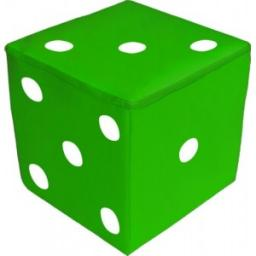Did you find an error or inaccuracy? Feel free to write us. Thank you!Tips to related online calculators
Would you like to compute count of combinations?

## Related math problems and questions:

• Roll the diceWhat is the probability that if we roll the dice, a number less than 5 falls?
• One threeWe throw two dice. What is the probability that max one three falls?
• DicesWe will throw two dice. What is the probability that the ratio between numbers on first and second dice will be 1:2?
• DiceWe throw five times the dice. What is the probability that six fits exactly twice?
• Three dice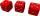What is the probability that when we roll three dice, we roll the numbers 1,2,3?
• Dice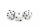How many times must throw the dice, the probability of throwing at least one six was greater than 90%?
• Sum 10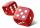What is the probability that two dice throw twice in a row will result the sum of 10?
• ThrowWe throw 2 times with 2 dices. What is the probability that the first roll will fall more than sum of 9 and the second throw have sum 3 or does not have the sum 4?
• Dice and coinA number cube is rolled and a coin is tossed. The number cube and the coin are fair. What is the probability that the number rolled is greater than 2 and the coin toss is head?
• Six on diceWhat is the probability that when throwing two dice will fall at least one six?
• Two diceWe roll two dice. What is the probability that the sum of the falling numbers is greater than 3?
• DiceWe throw 10 times a playing dice. What is the probability that the six will fall exactly 4 times?
• We rollWe roll two dice A. - what is the probability that the sum of the falling numbers is at most 4 B. - is at least 10 C. - is divisible by 5?
• Three dice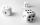When you throw three dice was the sum total of the dice 10. The yellow dice fell one eye more than on the red and brown fell 3 eyes less than red. How many eyes fell on every dice?
• Sum on diceWe have two dice. What is the greater likelihood of fall a total sum 7 or 8 ? (write 7, 8 or 0 if the probabilities are the same)?
• Dices throwsWhat is the probability that the two throws of the dice: a) Six falls even once b) Six will fall at least once
• The dice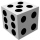Find the probability that a number divisible by two or three will fall when the dice are rolled.